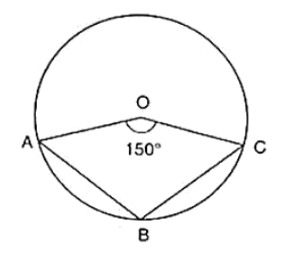# In figure, it is given that O is the centre of the circle and

Question:

In figure, it is given that O is the centre of the circle and ∠AOC = 150°. Find ∠ABC.Solution:

∠AOC = 150°

∴ ∠AOC + reflex ∠AOC = 360° [Complex angle]

⇒ 150° + reflex ∠AOC = 360°

⇒ reflex ∠AOC = 360° − 150°

⇒ reflex ∠AOC = 210°

⇒ 2∠ABC = 210° [By degree measure theorem]

⇒ ∠ABC = 210°/2 = 105°